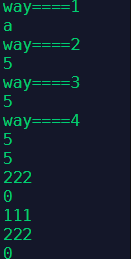本站每日ip已达10000,出租广告位,位置价格可谈,需要合作请联系站长

+关注

2019-08(58)

2019-09(95)

2019-10(18)

2019-11(2)

2019-12(17)

# 用法一

``````a, b = 5, 3
if a>b:
print("a")
else:
print("b")
```12345```

# 用法二

``````#一行表达式,if(1)return前者
#if(0)returnelse后面
c = a if a>b else b
print(c)
```1234```

# 用法三

``````#二维列表，利用大小判断的0，1当作索引
c= [b, a][a > b]
print(c)
```123```

# 用法四

``````#利用逻辑运算符进行操作
#利用and 的特点，若and前位置为假则直接判断为假。
#利用 or的特点，若or前位置为真则判断为真。
#如果a<b则and为0 or之后为[b]   c=([b])
#如果a>b则and为1 or之后不计算为[a]   c=([a])
c = (a>b and [a] or [b])
print(c)

# 改编版
c = (a>b and a or b)
print(c)

# 从前往后找，and找假，or找真
# 前真返后
print(111 and 222)  # 222
# 前假返前
print(0 and 333)  #0

print(111 or 222) #111
print(0 or 222) #222
print('' or 0) # 0

```12345678910111213141516171819202122```26 0